# Instantaneous center of rotation

In absence of any other forces, if you push a free object not on the center of mass, during the application of the force (not after) should it only rotate around its instantaneous center of rotation (also called pole or center of oscillation/percussion)? Or it can also be subjected to translational movement? And if that is case, considering that the axis of rotation is the pole, the only point that move lineary shouldn’t be the same pole?

Still when both linear and rotational movements are involved, even during the application of a force, I've always seen considering the body rotating around its center of mass, and in that case for what I know the linear force to which it’s subjected the center of mass is the same as the one applied, and the torque is equal to the force multiply the distance from the center of mass to the point of application of the force.

But if the point in translation is the pole then the linear force to which it’s subjected is still the same as the force applied?

For what I know it surely rotate around its center of mass only after the application of the force, but during it shouldn't. Is that right or wrong?

jbriggs444
Homework Helper
The same motion can be described either way. As a rotation around a motionless point - the instantaneous center of rotation. Or as a rotation around a different point along with a translation of that point.

Doing that doesn’t mean considering a tangential force as a linear force?
Because the arbitrary rotation point is not actually in translation. For small angle this can be a reasonable approximation but it shouldn’t be the same thing, right? So is it correct to consider only the module?

I made an image for explaining what i’m saying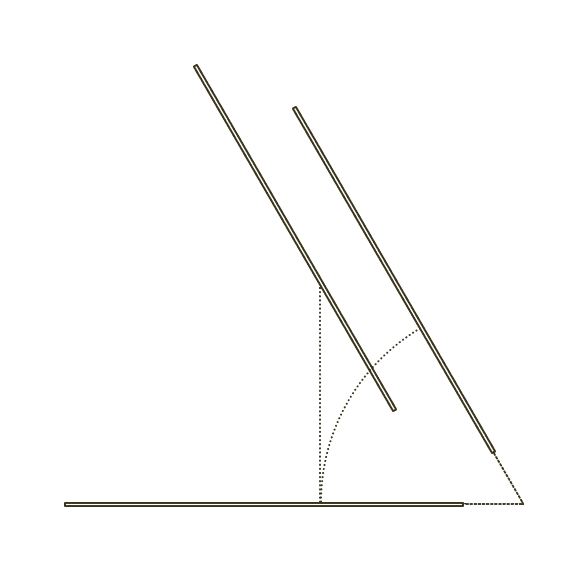#### Attachments

jbriggs444
Homework Helper
A force is a force, of course, of course. Tangential or not, a net force will result in a net change in linear momentum.

I think that I understand now what the diagram is attempting to depict.

You have the original stick position on the bottom. This stick is moving upward with the left end moving faster and the right end moving more slowly. The instantaneous center of rotation is at the point where the dotted lines intersect at the lower right.

There is an arbitrarily selected point of rotation that you have chosen to place about 2/3 of the way along the stick toward the right end. This is where the middle dotted line and the circular arc intersect.

Described as rotation about this arbitrary point plus translation of the point, the stick moves upward and is depicted at its new position above. The vertical dotted line depicts the motion of the selected point.

Described as rotation about the instantaneous center of rotation, the stick rotates up and to the right and is depicted at its new position, above and to the right. The curved dotted line depicts the motion of the arbitrarily chosen point.

That drawing is incorrect.

The thing about an instantaneous center of rotation is that it is an instantaneous center of rotation. If you check again an instant later, the figure may be rotating about a new and different instantaneous center of rotation.

Rotation about one fixed point is equivalent to rotation about a fixed point together with a translation only for an instant. That instant is the place on your drawing where the vertical dotted line and the curved dotted line intersect. For a moment they are tangent - at the same place and moving in the same direction.

Last edited:
The force F is applied at point A of an object.

In the first image the force makes the object rotate around the point C by an angle α.
In the second image the force makes the object rotate around the point B by the same angle α and makes the point B translate in the same direction of the applied force.

If the linear force that cause the translation of B in the second image has the same direction of the applied force F, I don’t see how C can remain stationary.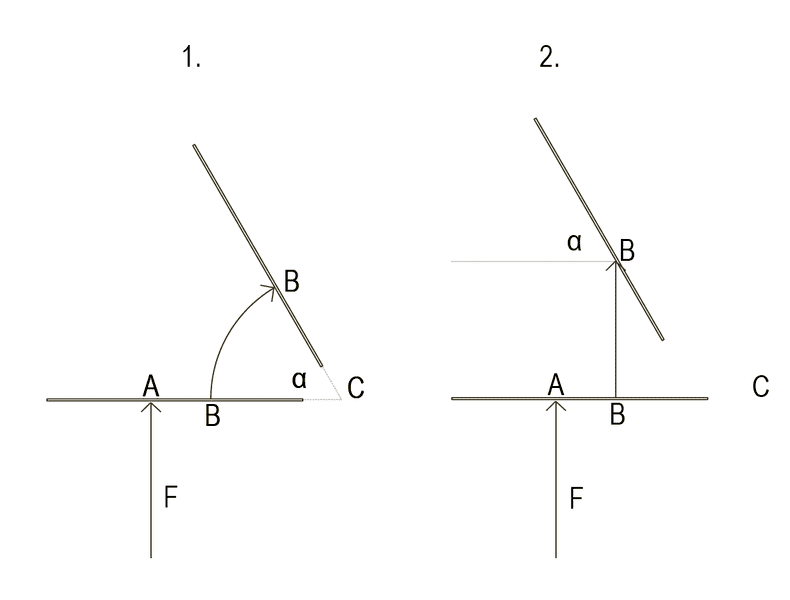The only way I can see it is if we consider B to be subject to a linear force with a module equal to the chord of tangential force in that point, and with a direction relative to the applied force equal to ((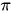/2) - α)/2 clockwise, plus a rotation around B of α+((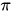/2) - α)/2 . But that should be true only for a given time frame, maybe infinitesimal.
I don’t know.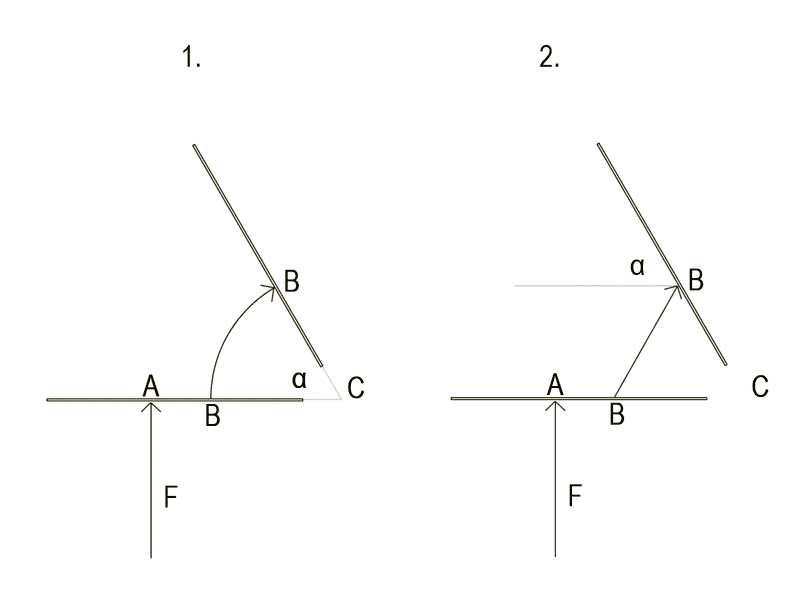Is there a demonstration of the fact that we can describe the motion of an object during the application of a force both by the rotation around a motionless point or by the rotation around a point that is also in translation?

#### Attachments

jbriggs444
Homework Helper
The force F is applied at point A of an object.

In the first image the force makes the object rotate around the point C by an angle α.
In the second image the force makes the object rotate around the point B by the same angle α and makes the point B translate in the same direction of the applied force.
As I wrote previously, the point C is the instantaneous center of rotation. If any time at all passes, the center of rotation need not stay there.

Stephen Tashi
Is there a demonstration of the fact that we can describe the motion of an object during the application of a force both by the rotation around a motionless point or by the rotation around a point that is also in translation?

The general nature of that question is interesting, but the question isn't specific.

If we make the question specific, I suspect the answer will be "No" because it can't be done.

In the first place, what is a "motionless" point? Technically, a point in a given coordinate system is a given location. It doesn't move with respect to the coordinate system.

In a given coordinate system when we talk about a "point" being in motion, we actually mean that there is some function P(t) = (x(t),y(t),z(t)) that describes a location that varies with respect to time. At a given time t0, P(t0) is a specific point. What would it mean to say that P(t) is "motionless"? Would that mean that P(t) is constant in time? - or only that P(t) has zero velocity at some time t0?

Doing that doesn’t mean considering a tangential force as a linear force?
What do you mean by a "tangential force"? What is it tangent to?

Since force is a vector, at a given instant of time, it has a magnitude and a direction. It isn't represented by a curve of some sort. What would a "nonlinear" force be? Do you wish to consider force that varies in magnitude or direction as time passes?

Consider the theoretical situation of a rod shaped space station "floating in free space".

Case 1) There is a rocket attached to the object (not at its center of mass). The body of the rocket cannot move with respect to the rod. The rocket exerts a constant thrust F that changes direction in time only due to the fact that the rod changes its orientation.

Case 2) There is a space "tug boat" the is moving along a straight line and has thrusters that can keep it moving along that line and in frictionless constant contact with the rod so the tugboat exerts a force of constant magnitude |F| against the rod

Case 3) There is a space "tug boat" that moves along some predetermined curved path and has thrusters that can keep it moving along that line an in frictionless contact with the rod so the tugboat exerts a force F(t) in a direction of non-constant magnitude |F(t)| against the rod such that the direction of F(t) is always tangent the curved path of the tugboat.

When you say "tangential force", do you refer to one of those three cases?

In the first place, what is a "motionless" point? Technically, a point in a given coordinate system is a given location. It doesn't move with respect to the coordinate system.

In a given coordinate system when we talk about a "point" being in motion, we actually mean that there is some function P(t) = (x(t),y(t),z(t)) that describes a location that varies with respect to time. At a given time t0, P(t0) is a specific point. What would it mean to say that P(t) is "motionless"? Would that mean that P(t) is constant in time? - or only that P(t) has zero velocity at some time t0?

Ok by definition the instantaneous center of rotation should have zero velocity in a given instant of time. So if I understood correctly it doesn’t moves only relative to the object, but it moves relative to an external fixed coordinate system in a way that can be described also with rotation around a given point of the object plus translation of that point (a centrode).

What do you mean by a "tangential force"? What is it tangent to?

Since force is a vector, at a given instant of time, it has a magnitude and a direction. It isn't represented by a curve of some sort. What would a "nonlinear" force be? Do you wish to consider force that varies in magnitude or direction as time passes?

Consider the theoretical situation of a rod shaped space station "floating in free space".

Case 1) There is a rocket attached to the object (not at its center of mass). The body of the rocket cannot move with respect to the rod. The rocket exerts a constant thrust F that changes direction in time only due to the fact that the rod changes its orientation.

Case 2) There is a space "tug boat" the is moving along a straight line and has thrusters that can keep it moving along that line and in frictionless constant contact with the rod so the tugboat exerts a force of constant magnitude |F| against the rod

Case 3) There is a space "tug boat" that moves along some predetermined curved path and has thrusters that can keep it moving along that line an in frictionless contact with the rod so the tugboat exerts a force F(t) in a direction of non-constant magnitude |F(t)| against the rod such that the direction of F(t) is always tangent the curved path of the tugboat.

When you say "tangential force", do you refer to one of those three cases?

The case I had in mind in general is the first.

But I used “tangential force” because I thought that it was possible to decompose a torque in the center of rotation (distance from center of rotation to point of application of the force multiplied the force) into a torque given by multiplying the distance between point of application and center of mass, plus the torque given by multiplying the distance between the center of mass and the center of rotation, which is maybe like saying that there is the same force applied in the center of mass. That should give something like a rotational and a translational component of the initial torque? But if it were the force applied in that specific point to make the object rotating around its center of rotation as a fixed point relative to a given coordinate system (which I get now it is not), then this decomposition of the torque should be valid only considering the module but not the vector of the hypothetical force in the center of mass. So I called as a tangential force a force of which we consider only the module since the vector changes during time, like a tangential acceleration or velocity. I’m not sure if that makes sense.

Thanks anyway.

jbriggs444
Homework Helper
Ok by definition the instantaneous center of rotation should have zero velocity in a given instant of time.
That depends on exactly what you mean by the velocity of the center of rotation.

If you think of the instantaneous center of rotation as a particular point fixed to your stationary coordinate system then it is also at rest with respect to a coordinate system nailed to the rotating object. If we have a wheel rolling on a road, the instantaneous center of rotation is located at the point where the wheel touches the road. For a brief instant, a pebble at that point on the road would be at rest both relative to the wheel and relative to the road. For a brief instant a piece of rubber on the tire would also be at rest both relative to the wheel and relative to the road.

However, if you wait a moment, the instantaneous center of rotation will not be there any longer. The point of contact will have moved on. The instantaneous center of rotation is no longer where it was before. If you follow the motion of the point of the point of contact, it has a velocity (equal to the velocity of the wheel in this case).

If you take the first notion for "velocity of the instantaneous center of rotation" then it is true that the velocity is zero. But the object only rotates around that point for an instant. A drawing that shows the object continuing to rotate around the same stationary point fixed to both object and ground is erroneous; the center of rotation, the place on the object which is both at rest relative to object and relative to the stationary frame, may be elsewhere.

Khashishi
I think you are overcomplicating this. A force applied to an object will change both the linear momentum and the angular momentum. The problem is that if the force is applied over an interval of time, the object will be moving during that interval of time, so in practice, where the force is applied will also probably change over time.

The change in the linear momentum doesn't depend on where the force is applied. If you push up off center, the object will still move up, assuming it is not attached to anything. However, if you push the end of a rod such that the rod starts to spin, you can only push the rod for an instant before it rotates away from your hand, making it difficult.

The change in angular momentum is just the torque. You can use any point of origin for the angular momentum and the equation will still work. But if a constant force is applied to a fixed point on an object, the object will move, so the torque will be changing in time relative to an origin that is not moving with the object. So, it may be convenient to use the center of mass frame which is a non-inertial reference frame since the center of mass is accelerating. Even though it's a non-inertial frame, it still works out for calculating the angular momentum about the center of mass.

Stephen Tashi